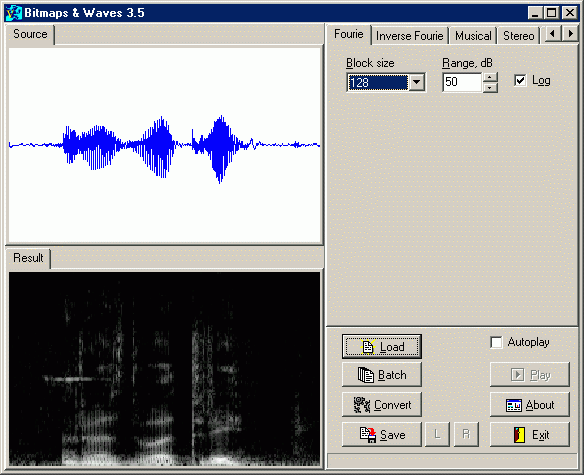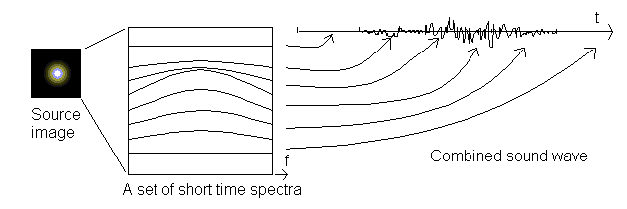Bitmaps & Waves Basics
Bitmaps & Waves is a program transforming pictures to sounds.
It provides the following methods to produce sound:
• inverse Fourier transform,
• superposition of signals with periodic or random (there are two different methods) instant frequency variations; the variation range is determined by the instant spectra.

The program can also transform sound to picture by means of direct Fourier transform and perform sound morphing through the morphing of pictures.

More details

The sound in a computer

Moving waves of changing air pressure are called sound. Sound we can hear is the pressure changes in frequency range from 20 to 20000 Hz (or cycles per second). A microphone transforms these pressure changes to voltage. At this step, signal (voltage) is continuous, also called analogous, i.e. it has certain values at every instant. Analog-to-Digital Conversion (ADC) transforms analog signal to the discrete one, i.e. a signal represented as a sequence of numeric values in certain time moments. ADC is a component of all sound cards installed in computers. ADC measures voltage on its input connector in a time interval called sampling period. The number of measurements per second is called sampling rate. The results of the sampling (numeric voltage values) are stored in the memory of a computer. It is obvious that correct storage of high frequency signals needs higher sampling rate. This relationship is described by Nyquist theorem, which proves that sampling rate must be at least two times higher than the highest frequency component of the signal. For example, audio CD stores sound in digital representation, and the sampling rate 44100 Hz is used. This gives the possibility to store non-distorted sounds in a frequency range up to 20000 Hz.

Sound signal spectrum

We know that sounds have different pitches. There are low-frequency (bass) sounds and high-frequency (treble) sounds. Musical instruments, like other sound sources, radiate sounds superposed on one another. The result is a bizarre mix, sometimes called music. We can, however, identify separate sounds... There is a "built-in" set of filters in the ear and each of the filters extracts signal in the narrow frequency range. This can also be done by a program using Fourier transform. The transform decomposes signal to harmonic components with pure frequencies. Input data for the transform is a set of numeric values of a sound's signal - voltage at the ADC input connector. Output data is an amplitude spectrum of signal - component's amplitudes as function upon its frequency, and a phase spectrum of signal - a set of numbers showing the relative time shift of components.

Usually Fourier transform is performed by means of Fast Fourier Transform (FFT) algorithm. FFT requires the number of input values to be a power of 2, i.e. 2, 4, ... 512, 1024, ... 65536, ... Assume that the input for Fourier transform contains values of a sine wave. In that case output amplitude spectrum contains the only nonzero value at the frequency of the input sine wave. If we change the frequency of the input wave, the nonzero value in the amplitude spectrum will be found at another place. If the input array is filled with the sum of two sine waves with different frequencies, the output array contains two nonzero values, etc.
However, nothing lasts forever in nature. Sounds appear and disappear, or change in time. Thus, spectra of different parts of a real sound signal may be different, and such a signal is called non-stationary. For convenience, changing spectra are often displayed as a spectrogram - an image, each line of which represents the instant spectrum of a certain part of a signal. Different colors correspond to different amplitude values; usually black color corresponds to zero amplitude.Look at the picture above. The spectrogram is computed from a sound of words "one two three" pronounced by the author in Russian. The beginning of the signal is at the left side of the picture. You can make a spectrogram of any signal: In BW select the Fourie tab and load a wave file (such files have extension ".wav" in MS Windows) - it may have been created with Sound Recorder or a more sophisticated sound editor. Choose left or right channel if the file contains stereo signal. Press the Convert button. Wait a little, and the spectrogram appears. It is interesting to use musical instruments to produce sounds of different pitch and find out the difference in spectrograms.

Sound synthesis by means of inverse Fourier transform

However, Fourier transform has another remarkable feature - it is reversible. So we can not only calculate signal spectrum, but create signal with given spectrum too. This is called inverse Fourier transform. Let's get back to our task - the creation of sound from picture. Assume the source picture is a spectrogram of the sound signal to be produced.Consider each line of the image as an amplitude spectrum of sound signal. Assume that brightest points of the image correspond to the greatest spectral values, and the darkest ones - to the lowest. Color is not taken into account, but brightness only. Performing of inverse Fourier transform for all lines of the source image produces a sound signal. Notice that the program starts conversion from the left side of the image. If the resulting signal will be converted back to a picture (use Fourie tab to perform such conversion), this picture would be similar to the source image. Here is another field for experiments. Use any image editor - such as MS Paint or more sophisticated Adobe PhotoShop - to draw something. For example, white diagonal line on black background. Save the image as a .bmp (Windows bitmap) file and load it from the main window of BW. Such a picture will produce a sound with sweeping frequency. One day you can create a symphony after adding more elements to the picture.
Another method of synthesis can give you different sounds.

Sound synthesis by superposition of signals with varying instant parameters

Inverse Fourier transform produces noise from a white rectangle. Every wide painted area produces noise. Sometimes this is not desired. How can the signal with a frequency varying in a given range be produced? The solution can be obtained from two alternative methods of sound synthesis of pictures. Both of them extract separate objects in a picture assuming that the background is black. Every extracted object produces sound with a duration dependent on horizontal object dimension, while the frequency range of sound depends on width and vertical position of the object. Instant frequency varies from low to high limits of that range with a certain period in the method called Vibrations. Another method named Threads produces sound with instant frequency varying in the same range using random function. Brightness of the object is taken into account too. For example, if the upper edge of the object is brighter than the lower one, high frequency sounds will have greater amplitude than low frequency sounds. Turning the switch Show Objects on, we can see instant values of sound pitch drawn over the objects while using the method Vibrations. The Threads method shows the edges of extracted objects. Unlike inverse Fourier transform, these methods use the colors of objects if the switch Use colors is on. Besides the main tone, one or two additional sounds are produced to obtain a chord. If the color of the object is warm it would be a major chord, else (if the color is cold) a minor chord. If the color is used for synthesis, the switch Show Objects is always off.

Morphing

Sound morphing is a sound synthesis from two source sounds. The result possesses features of both of them. The most interesting is the creation of a hybrid with varying degrees of the sources' influence. BW performs picture-based morphing. To try it, select the Morphing tab. Load two images, then press the Convert button. The influence of the sources can be adjusted by mouse clicking at Hybrid signal contents plot, or corresponding numeric values between 0 and 1 can be entered into the table (press Apply button to see the changes on the plot above).
There are two methods of image combining - addition and multiplication. The first uses weighted average values to calculate the brightness of each pixel of the result image. The second method multiplies values of source brightness. If one source point is black, and another is white, the first method produces gray, and the second produces black (multiplication by zero brightness produces zero). Such image-based implementation of sound morphing produces specific distortion but to the loss of phase spectrum components. This method, however is more demonstrative than others and good enough for home use.

Samples: